# Introduction to atpolR

The ATPOL grid was created in the late 1960s at the Institute of Botany at Jagiellonian University in Kraków. (Zając 1978a, 1978b) describes the background and methodology. Łukasz Komsta and Marek Verey carried out the extensive mathematical research and GIS implementation (Komsta 2016; Verey 2017). Algorithms provided by Komsta on OpenATPOL are basis for implementation in atpolR package.

# Basic usage

## Prepare sample data based on published ATPOL data

In our example we’ll use a distribution map of Erigeron acris L. subsp. acris from (Zając and Zając 2019). The image was scanned at 150 dpi and georeferenced with QGIS.

``````par(mar = c(0, 0, 0, 0))
tif <- system.file("extdata/eriacr.tif", package = "atpolR")
r <- terra::rast(tif)
terra::plotRGB(r)``````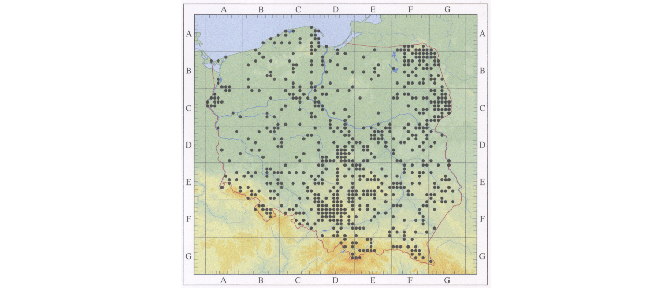Erigeron acris L. subsp. acris distribution taken from (Zając and Zając 2019)

There is hundreds of records. To get them all, we will use `check_atpol_square()` function, which takes the POINT coordinates and a raster as arguments and checks if the value of raster cell corresponding to some arbitrary buffer around the POINT equals zero. As the points are drawn in centers of ATPOL 10 km grid, the values of atpol10k() centroids with an buffer with radius of 1200 m will be checked. It may be necessary to adjust a buffer slightly depending of the quality of scan and precision of georeferencing.

The raster usually consist of 3 layers, one for each R, G, B component. The difference between them are visible on Fig. @ref(fig:rgbraster).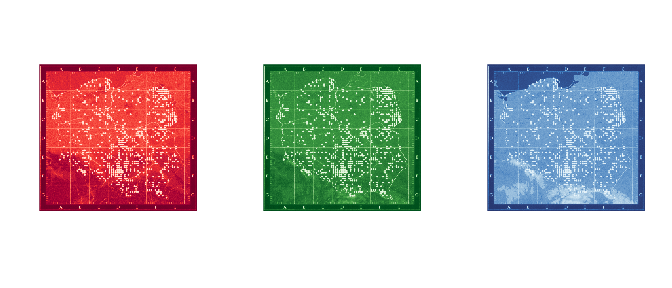R, G and B layers of a raster

We can see a lot of green and blue components in the scan shown in Fig. @ref(fig:eriacr). We will only look at the first layer for now. Please keep in mind that other layers may be more useful depending on the scan quality and subsequent image processing.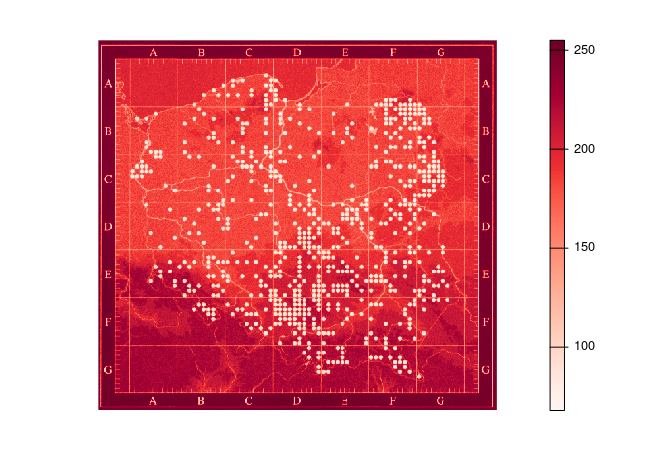The layer’s values are continuous, ranging from 0 to 255. To simplify and facilitate analysis, we will classify the layer by assigning only two values: 0 and 1. We’ll make a reclassification matrix called `rclmat` and use the `classify()` function form the terra package.

``````m <- c(0,120, 0,
120,255, 1)
rclmat <- matrix(m, ncol=3, byrow=TRUE)
rc <- terra::classify(r[], rclmat, include.lowest = TRUE)``````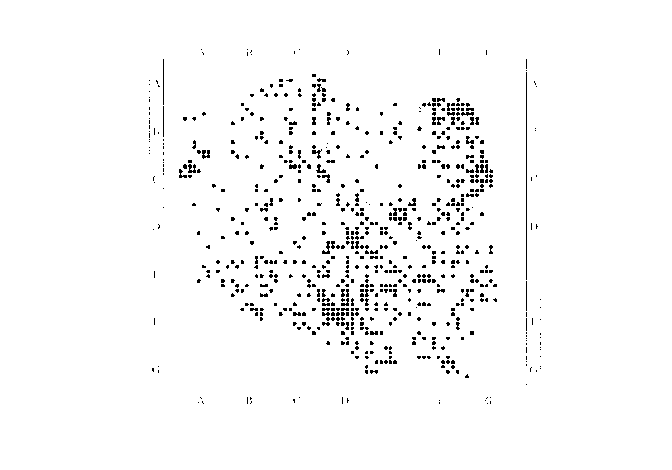Reclasiffied raster with 0 — as black and 1 — as white

After preparing the raster, we can load the package and run the `check_atpol_square()` function for all 10k grids:

``````library(atpolR)

eriacr <- atpol10k()|>
dplyr::mutate(a = mapply(function(x) check_atpol_square(x, rc), centroid)) |>
dplyr::filter(a == "YES")``````

Let’s display the results:

``````eriacr
#> Simple feature collection with 709 features and 2 fields
#> Active geometry column: geometry
#> Geometry type: POLYGON
#> Dimension:     XY
#> Bounding box:  xmin: 170032.6 ymin: 140353.6 xmax: 839912.3 ymax: 778164.1
#> Projected CRS: ETRS89 / Poland CS92
#> First 10 features:
#>    Name                       geometry                  centroid   a
#> 1  AB15 POLYGON ((220203.9 698774.2... POINT (225196.1 693780.2) YES
#> 2  AB21 POLYGON ((180184 688879.5, ... POINT (185177.4 683882.5) YES
#> 3  AB23 POLYGON ((200188.2 688840.5... POINT (205181.3 683844.9) YES
#> 4  AB73 POLYGON ((200121.5 638968.2... POINT (205117.5 633972.6) YES
#> 5  AB83 POLYGON ((200110.3 628991.5...   POINT (205106.9 623996) YES
#> 6  AB94 POLYGON ((210103.7 619001, ... POINT (215100.6 614006.2) YES
#> 7  AB95 POLYGON ((220106.8 618988.3... POINT (225103.5 613994.1) YES
#> 8  AB96 POLYGON ((230109.2 618976, ... POINT (235105.7 613982.3) YES
#> 9  AC05 POLYGON ((220097.7 609011.9... POINT (225094.9 604017.7) YES
#> 10 AC06 POLYGON ((230100.4 609000.3... POINT (235097.4 604006.7) YES``````

There is 709 observations (grids) in data set.

Let’s have a closer look on BE square:

``````BE <- atpol100k() |>
subset(Name == "BE") |>
sf::st_bbox()
par(pty = "s")
plot(NA, type = "n", xlim = c(BE, BE), ylim = c(BE, BE), axes = FALSE, xlab = "", ylab = "")
terra::plot(rc, legend = FALSE, add = TRUE)

atpol100k() |>
subset(Name == "BE") |>
sf::st_cast("LINESTRING") |>
terra::plot(add = TRUE, col = "blue", lwd = 1.2)

eriacr |>
subset(substr(Name, 1, 2) == "BE") |>
sf::st_set_geometry("centroid") |>
terra::plot(pch = 16, cex = 1.2, col = "blue", add = TRUE)``````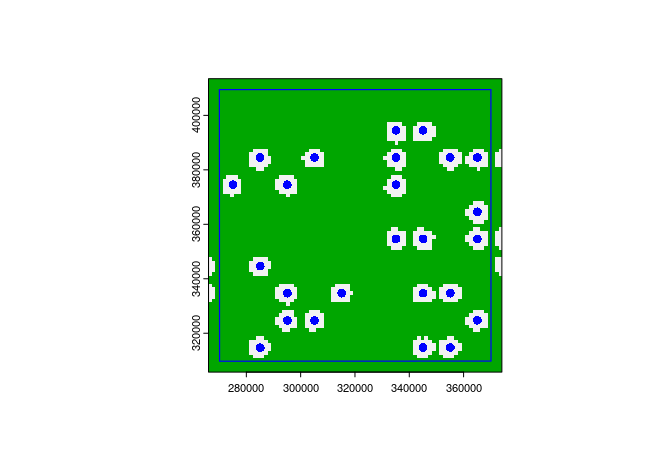Thus far, so god. Our data set corresponds to published data. In the next step we will add our own observations.

Please keep in mind that `check_atpol_square()` function can produce false positive results in the case of noisy scans. it also does not recognize the various shapes used in original publications for various sites.

## Extend the data

Assume we want to add our own observations the data set. If we already know the grid name, we can simply use the Name to filter out the grid created by the `atpol10k()` function. We can use `latlon_to_grid()` function if we don’t have the grid but have coordinates. In the following example, we are using both methods:

``````myData <- atpol10k() |>
dplyr::filter(Name %in% c("BE68",
latlon_to_grid(51.13619, 16.95069, 4))) |>
dplyr::mutate(a = "myData")``````

And let’s add them to above BE square plot:

``````myData |>
sf::st_set_geometry("centroid") |>
terra::plot(pch = 16, cex = 1.8, col = "red", add = TRUE)``````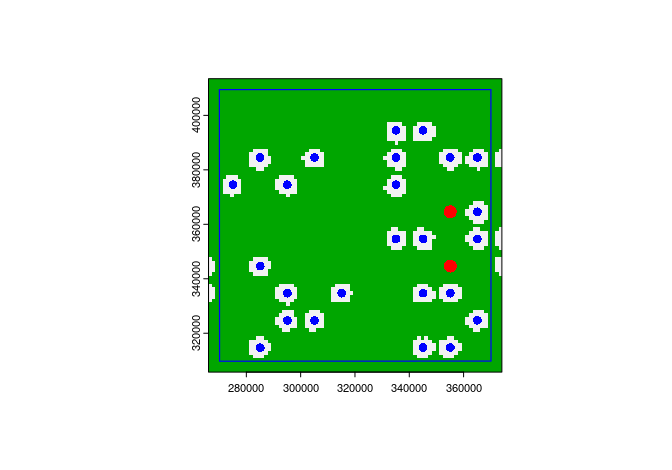Data set extended with our observations in grids BE48 and BE68

## Plot it

atpolR package provides a function `plot_points_on_atpol()` which can be used to visualize the data set. Let’s merge our two data sets (`eriacr` and `myData`) and plot them together. For removing any duplicates we can use `unique.data.frame()` function from base R, or `distinct(Name)` from dplyr package.

``````eriacr <- eriacr |>
rbind(myData) |>
unique.data.frame()``````

And final plot.

``plotPoitsOnAtpol(eriacr\$centroid, main = "Erigeron acris subsp. acris", cex = 0.6)``